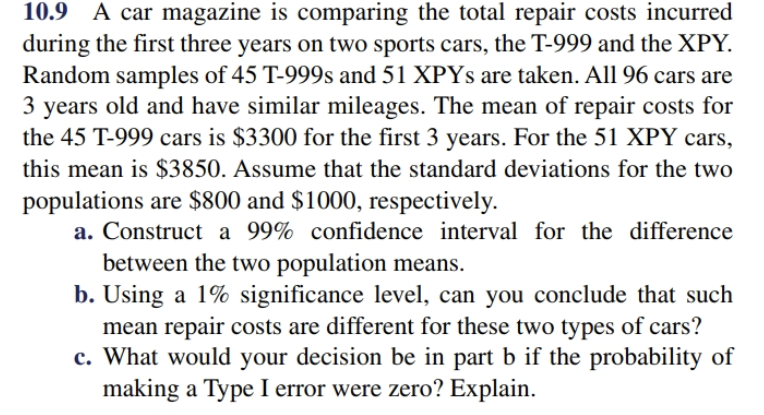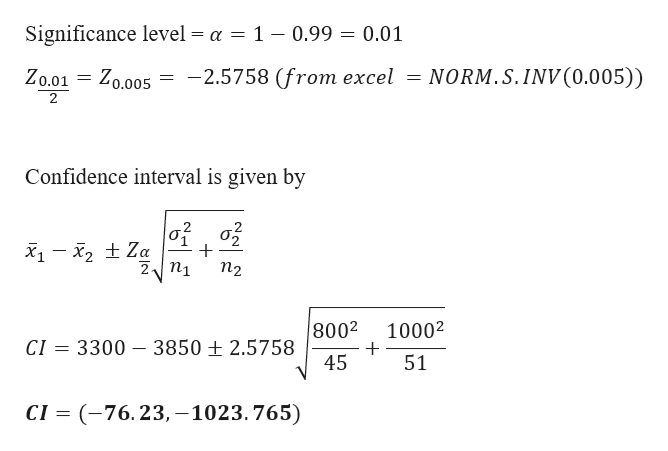# 10.9 A car magazine is comparing the total repair costs incurredduring the first three years on two sports cars, the T-999 and the XPY.Random samples of 45 T-999s and 51 XPYS are taken. All 96 cars are3 years old and have similar mileages. The mean of repair costs forthe 45 T-999 cars is \$3300 for the first 3 years. For the 51 XPY carsthis mean is \$3850. Assume that the standard deviations for the twopopulations are \$800 and \$1000, respectivelya. Construct a 99% confidence interval for the differencebetween the two population meansb. Using a 1% significance level, can you conclude that suchmean repair costs are different for these two types of cars?c. What would your decision be in part b if the probability ofmaking a Type I error were zero? Explain

Question
60 viewshelp_outlineImage Transcriptionclose10.9 A car magazine is comparing the total repair costs incurred during the first three years on two sports cars, the T-999 and the XPY. Random samples of 45 T-999s and 51 XPYS are taken. All 96 cars are 3 years old and have similar mileages. The mean of repair costs for the 45 T-999 cars is \$3300 for the first 3 years. For the 51 XPY cars this mean is \$3850. Assume that the standard deviations for the two populations are \$800 and \$1000, respectively a. Construct a 99% confidence interval for the difference between the two population means b. Using a 1% significance level, can you conclude that such mean repair costs are different for these two types of cars? c. What would your decision be in part b if the probability of making a Type I error were zero? Explain fullscreen
check_circle

Step 1

Given data
Car T-999

Step 2

a) 99% Confidence interv...help_outlineImage TranscriptioncloseSignificance level = a = 1 - 0.99 = 0.01 -2.5758 (from excel = NORM.S. INV (0.005)) Zo.01 Zo.005 2 Confidence interval is given by |010물 12 Za 2n1 П2 8002 10002 2.5758 CI 3300 3850 45 51 СI 3 (-76. 23, -1023.765) fullscreen

### Want to see the full answer?

See Solution

#### Want to see this answer and more?

Solutions are written by subject experts who are available 24/7. Questions are typically answered within 1 hour.*

See Solution
*Response times may vary by subject and question.
Tagged in

### Other